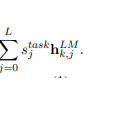# Softmax normalized weights in ELMO

I was going through the ELMo paper, here is the link :- https://arxiv.org/pdf/1802.05365.pdf
It has softmax normalized weights multiplied ashere s is the softmax normalized weights and h is the output of BiLSTM jth layer and for kth token.
as this ‘s’ is task-specific it is to be learned, my question is that what is the most pytorchy way to do it, if we want to implement it from scratch?